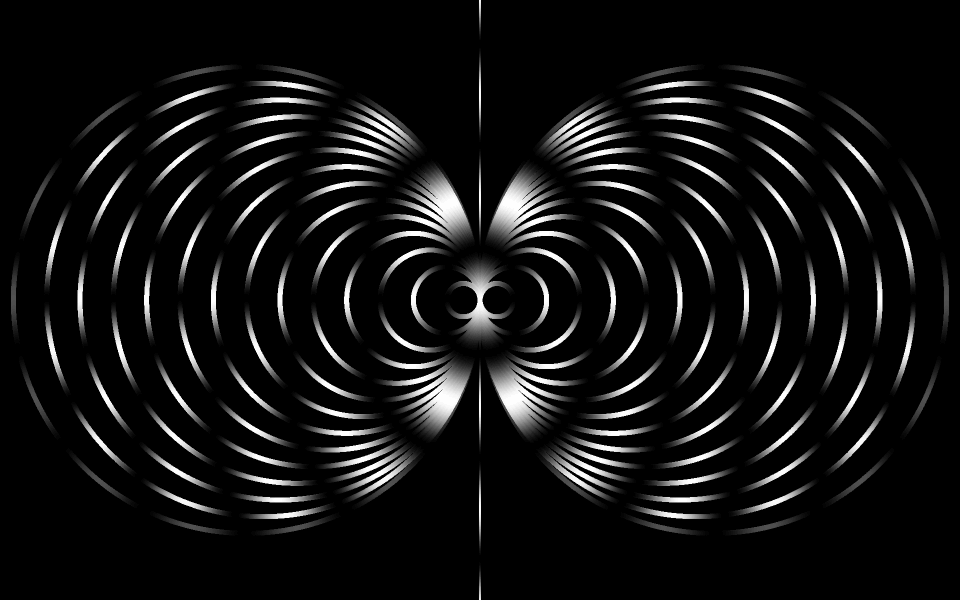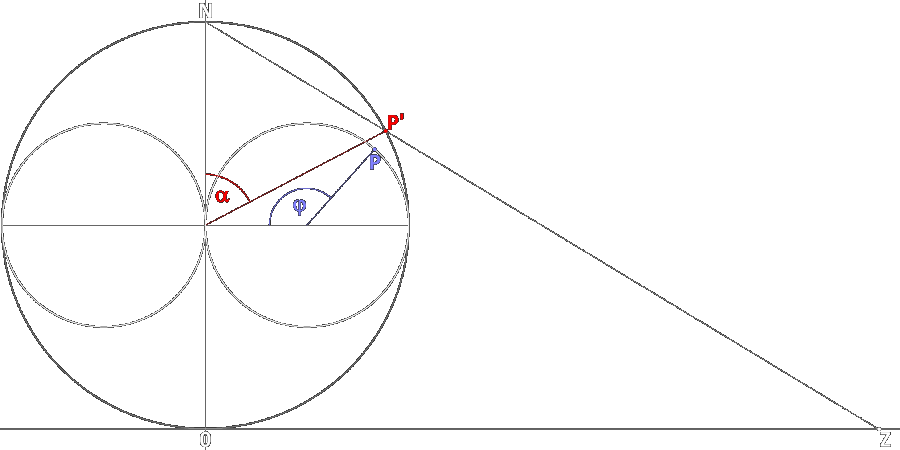conformal mapping of point P' on a sphere to point P on a horn torus, equivalent to  α → φ,when choosing α = 0 at the north pole  (later we change to version with α = 0 at south pole, which is symmetric, but more logical and consistent, because |z| is zero here simultaneous)φ = 2·arccot(-ln(|tan(α/2)|) - C)we observe a small circle around P' on sphere with radius dα   (α is latitude, 0 at north pole) the radius perpendicular to screen in P' with same length is dω = dα / sin(α) (ω is longitude) the projected small circle around P on horn torus has same radius dω  (as angle, not length) and for corresponding perpendicular radius dφ we respectively have:  dω = dφ / (1 - cos(φ)) ω and dω, as angles, are identical on both figures, therefore:    dα / sin(α) = dφ / (1 - cos(φ)) by integration and solving for φ we get above result values for α:  0 to π, resulting values for φ:  0 to 2·π approximations to both limits are highly asymptotic and absolute symmetrical from both sides of center * to get φ < 4° on horn torus, α has to be < 10^(-10)°, to get φ < 3° on horn torus, α has to be < 10^(-14)°, to get closer than 2° - my computer's capacity fails!  (α < 10^(-26)) an extremly slow approach to the horn torus center reminds me of conditions at the edge of black holes and of the different views ahead and backwards, when riding a photon - with speed of light:   []   ⇐   previous page  current version  •  even slower 'approach' when mapping direct from the complex plane  (α = 2·arccot(|z|))  •  latter inserted into above formula we eventually get:   φ = 2·arccot(-ln|tan(arccot(|z|))|)  •  inverse mapping from horn torus to complex plane: |z| = cot(π/2 - arccot(e^(-cot(φ/2))))  •  inverse mapping from horn torus back to Riemann sphere: α = π - 2·arccot(e^(-cot(φ/2)))  •  most likely no stereographic projection possible, but mathematicians surely will look for!  •  non-conformality creates pattern in the dynamic model, but conformality has a meaning   *  we obtain much simpler formulas when we determine angle α to be zero at south pole 0 my rough cross-checks with various angles α and differentials Δω indicate exact validity, but analytical evidence and search for a projective geometry  I gladly surrender to professionals  ...   homeRiemann sphere:  0N = 1 , |z| = 0Z ,  ∠0ZN  =  ∠N0P'  =  α/2
(1/sin(α))dα  =  (1/(1 - cos(φ)))dφ    ln(|tan(α/2)|)  =  - cot(φ/2) + C    α  =  2·arctan(e^(-cot(φ/2) + C))    φ  =  2·arccot(-ln(|tan(α/2)|) - C)   (C any real number)    X